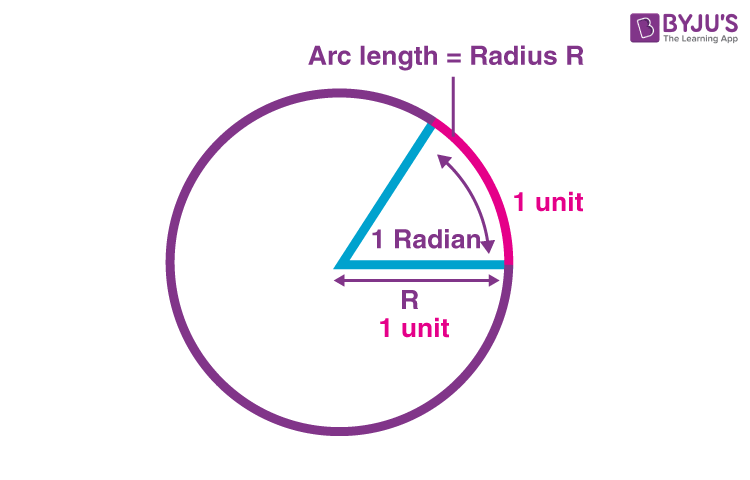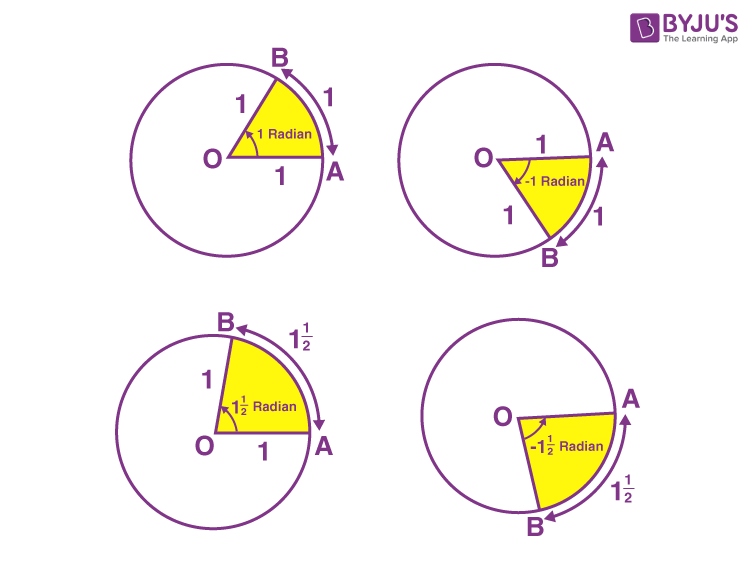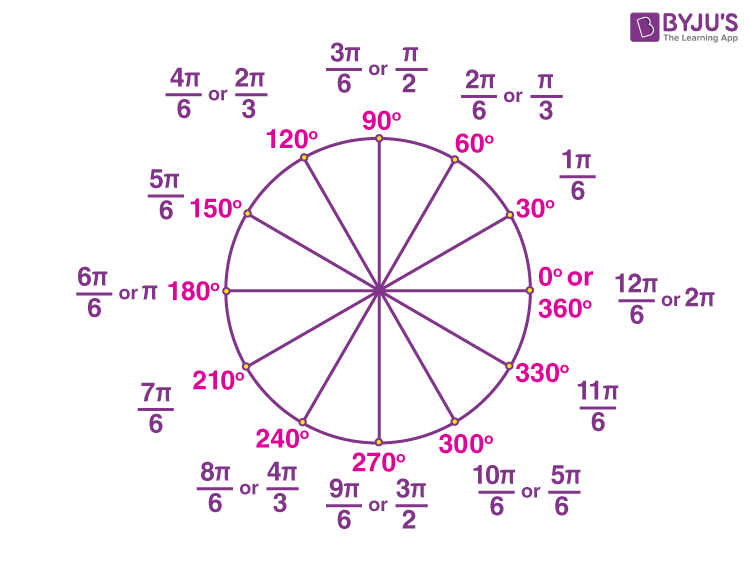In measuring angles, we generally use two types of measuring units, namely degrees and radians. Both measurements are widely used units in geometry and trigonometry in solving many problems. In general, the degree measure is used in earlier classes of mathematics since it is easy to understand and remember as this involves use of a protractor to measure the angle. In case of radians, one should understand the concept of circles and related terms along with their meaning and formulas. In this article, what is the radian measure of an angle, how to find the measure of an angle in radians and measurement of different angles in radians are explained.

Angle subtended at the centre by an arc of length 1 unit in a unit circle (circle of radius 1 unit) is said to have a measure of 1 radian. The symbol used to denote the radian measure is “rad” or “c”. This is shown in the figure given below.The circumference of a circle of radius 1 unit is 2π since the circle’s circumference with radius “r” is 2πr.

Thus, one complete revolution of the initial side subtends an angle of 2π radians.

Further, in a circle of radius r, an arc of length r will subtend an angle of 1 radian.

As we know, equal arcs of a circle subtend equal angles at the centre. Considering a circle.

Arc length = r

From this, we can say that an arc of length l will subtend an angle whose measure is l/r radian.

Let r be the radius of a circle, l be its arc length subtends an angle θ radian at the centre. Thus,

θ = l/r

Or

l = r θ

### Radian measure of an Angle### Radian Measure and Degree Measure

We know that the measurement of an angle can be performed in either of the measures, i.e. radian measure or degree measure. Also, we can define a relation between these two so that we can convert one unit of measurement to another, i.e. from radian measure to degree measure and vice versa.

1 radian = 180°/π = 57° 16′ (approx)

Using the above relations, let’s convert a few angles. These are useful to learn how to find the radian measure for any given angle.

We know that,

25° = 25 × (π/180)

Or

We know that,

240° = 240 × (π/180)

Or

We know that,

520° = 520 × (π/180)

Or

Unlike degree measures, it is possible to represent the radian measure of an angle using a circle. The below figure shows the radian measure of some specific angles.Go through the solved examples given below.

Example 1:

Which radian measure is equivalent to -15 degrees?

Solution:

We know that,

-15° = -15 × (π/180)

Therefore, -0.2617993878 rad is equivalent to -15 degrees.

Example 2:

Which radian measure is equivalent to 75 degrees?

Solution:

We know that,

75° = 75 × (π/180)

Therefore, 1.308996939 rad is equivalent to 75 degrees.

Example 3:

How many radians is 90 degrees?

Solution:

As we know, one complete revolution = 2π radians or 360°